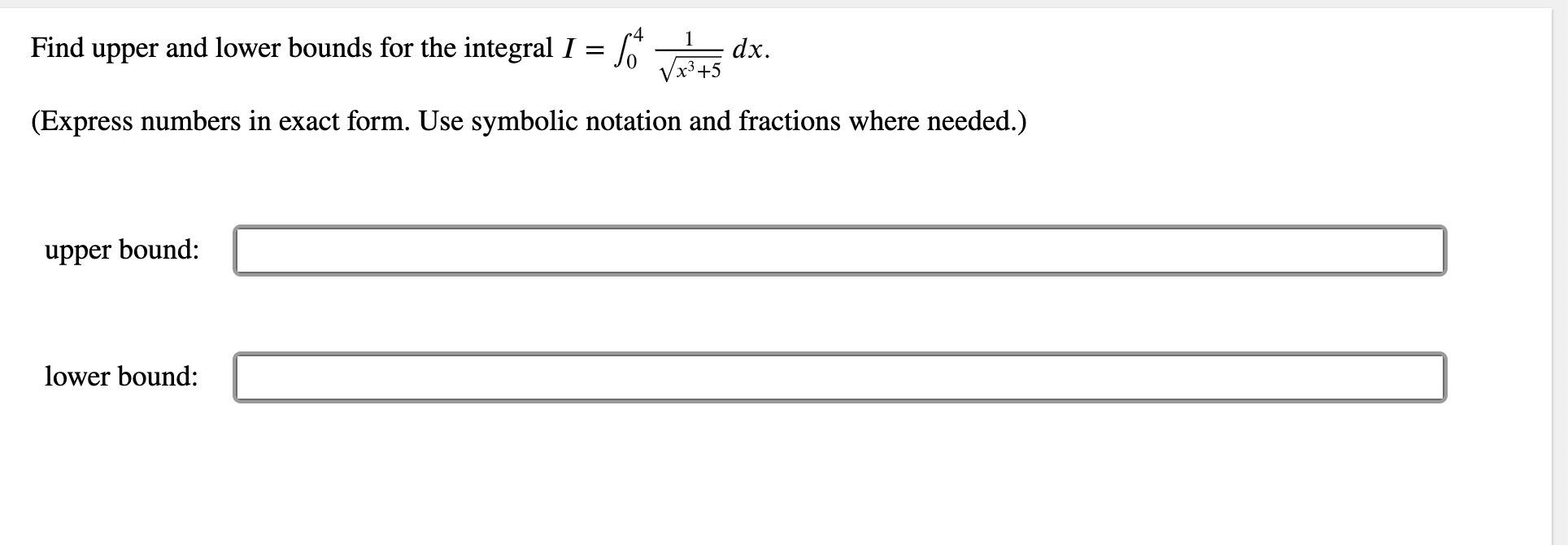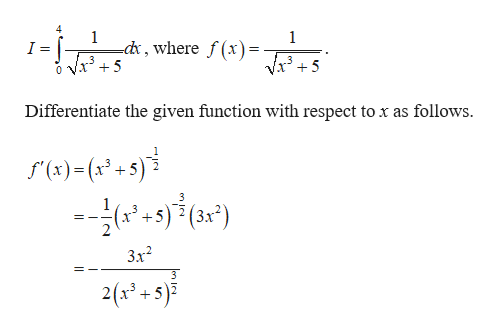# 1Find upper and lower bounds for the integral I =dx.x3+5(Express numbers in exact form. Use symbolic notation and fractions where needed.)upper bound:lower bound:

Question
23 viewshelp_outlineImage Transcriptionclose1 Find upper and lower bounds for the integral I = dx. x3+5 (Express numbers in exact form. Use symbolic notation and fractions where needed.) upper bound: lower bound: fullscreen
check_circle

Step 1

The given integral is,help_outlineImage Transcriptionclose4 1 1 cd, where f(x) I = Differentiate the given function with respect to x as follows. f'(x)= (x3 +5) 2 3 3х? 2(x 2+5)3 fullscreen
Step 2

Compute the criti...

### Want to see the full answer?

See Solution

#### Want to see this answer and more?

Solutions are written by subject experts who are available 24/7. Questions are typically answered within 1 hour.*

See Solution
*Response times may vary by subject and question.
Tagged in

### Other